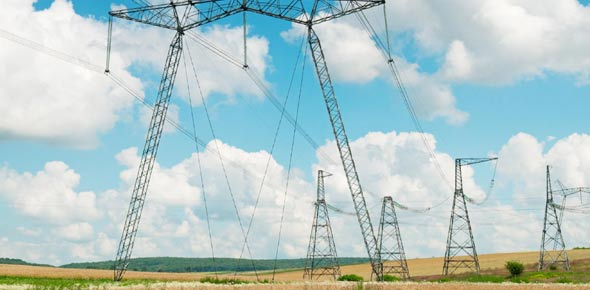# Electricity

24 QuestionsSettings.

Related Topics
• 1.
When an object is positively or negatively charged, it has ______ electricity
• 2.
Positively charged objects have ____ electrons, negatively charged objects have ______ them
• A.

Lost, gained

• B.

Gained lost

• 3.
Charged objects attract neutral (uncharged) objects
• A.

True

• B.

False

• 4.
Charge can be produced by fr______
• 5.
Negatively charged objects discharge if touched by a conductor - like a metal or a person. This is because electrons like to move through these - they want to get to earth
• A.

True

• B.

False

• 6.
Lightning and sparks are caused by a discharge of electrons between positive and negative regions, or when the electrons 'jump' between a charged object and conductor.
• A.

True

• B.

False

• 7.
What's the following apparatus?
• A.

Electrical ball

• B.

Electrical generator

• C.

Van De Graaff generator

• D.

Power ball

• 8.
This shows the stages of how a ___________ works
• 9.
What happens to the CURRENT in a SERIES circuit
• A.

It stays the same throughout

• B.

It changes depending on equipment

• C.

It usually stays the same

• D.

Is usually changes

• 10.
For a PARALLEL circuit, the current that leaves is the SAME as the current that returns. Current divides into half when it has 2 routes but it eventually meets in the end
• A.

Yep

• B.

Nope

• 11.
Voltage is a measure of how big of a push the electrons are given as they leave the cell of battery, can also be thought of as energy
• A.

True

• B.

False

• 12.
Select some things which can produce the voltage
• A.

Cell

• B.

Lamp

• C.

Battery

• D.

Power supply

• 13.
How does voltage work in a series circuit?
• A.

The sum of voltages across each component add up to the voltage across the entire battery

• B.

The voltage is the same throughout

• 14.
For a PARALLEl circuit, the VOLTAGE across the battery is the same as the voltage across each branch
• A.

True

• B.

False

• 15.
What does Ohm's Law tell us?
• A.

As the voltage increases, the current also increases - proportionally

• B.

As the voltage increases, the current decreases - proportionally

• C.

Technically, its none of the above

• 16.
In general, for a series circuit, what's the total resistance, R_T?
• A.

R_T = (R_1) * (R_2) * (R_3) * ... * (R_n)

• B.

R_T = (R_1) / (R_2) / (R_3) / ... / (R_n)

• C.

R_T = R_1 + R_2 + R_3 + ... + R_n

• D.

1/(R_T) = 1/(R_1) + 1/(R_2) + 1/(R_3) + ... + 1/(R_N)

• 17.
In general, for a parallel circuit, whats the total resistance R_T?
• A.

R_T = R_1 + R_2 + R_3 + ... + R_n

• B.

1/(R_T) = 1/(R_1) + 1/(R_2) + 1/(R_3) + ... + 1/(R_n)

• C.

R_T = (R_1) * (R_2) * (R_3) * ... * (R_n)

• D.

R_T = (R_1) / (R_2) / (R_3) / ... / (R_n)

• 18.
Calculate the total resistance. Leave your answer as a fraction. Your answer is in Ω (Ohms). (Here's a hint: 1/(R_t) = 1/12 + 1/18 + 1/6 = 3/36 + 2/36 + 6/36)